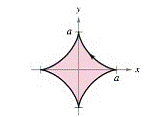Chapter 10.3, Problem 82E

Chapter
Section
Textbook Problem

Area of Simple Closed Curves In Exercises 81-86, use a computer algebra system and the result of Exercises 77 to match the closed curve with its area. (These exercises were based on “The Surveyor’s Area Formula” by Bart Braden, College Mathematics Journal , September 1986, pp335-337, by permission of the author.)(a) 8 3 a b (b) 3 8 π a 2 (c) 2 π a 2 (d) π a b (e) 2 π a b (f) 6 π a 2 Astroid :     ( 0 ≤ t ≤ 2 π ) x = a cos 3 t y = a sin 3 tTo determine

To calculate: The close curve area of an astroid, x=acos3t and y=asin3t in the interval 0t2π by the graphing utility and match the result with the given areas.

Explanation

Given:

Parametric equations are: x=acos3t,y=bsin3t and the interval, 0t2π.

Formula used:

If y is a continuous function of x on the interval axb, where x=f(t) and y=g(t), then,

|abydx|=|t1t2g(t)f(t)dt|, where f(t1)=a and f(t2)=b and both g and f are continuous on [t1,t2].

Calculation:

Consider the equation of the asteroid, x=acos3t,y=asin3t.

Assume that f(t)=x and g(t)=y.

Now use the formula, |abydx|=|t1t2g(t)f(t)dt| to find the area of the asteroid,

So, differentiate f(t) with respect to t

Still sussing out bartleby?

Check out a sample textbook solution.

See a sample solution

The Solution to Your Study Problems

Bartleby provides explanations to thousands of textbook problems written by our experts, many with advanced degrees!

Get Started

In Exercises 5-8, graph the given function or equation. 2x3y=12

Finite Mathematics and Applied Calculus (MindTap Course List)

Define the terms population and sample, and explain the role of each in a research study.

Essentials of Statistics for The Behavioral Sciences (MindTap Course List)

Conic What conic section does the polar equation r a sin + b cos represent?

Calculus: Early Transcendental Functions (MindTap Course List)

In Exercises 1-8, construct a truth table for each compound proposition. ~p~q

Finite Mathematics for the Managerial, Life, and Social Sciences

True or False: converges.

Study Guide for Stewart's Multivariable Calculus, 8th